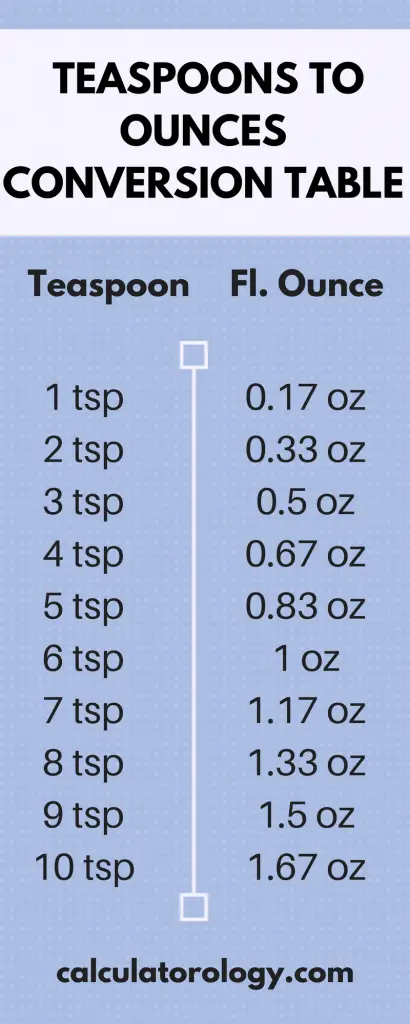## Teaspoons to Ounces Conversion

Converter

Enter a value that you want to convert into US fluid ounces and click on the "convert" button.

### Teaspoon to Ounces (How many ounces in a teaspoon?)

A teaspoon is a volume unit that is often used in cooking recipes and prescriptions. There are various types of teaspoons that are used as units of measurement and are available in different sizes. One US teaspoon is equivalent to 1/6 of fluid ounces and 5 milliliters. It is usually abbreviated as "tsp" or "ts". A teaspoon is usually abbreviated in small letters to avoid it being confused with a larger volume unit i.e. a tablespoon. A fluid ounce is a US customary and an imperial volume unit. An ounce is different from fluid ounce because it is a mass (weight) unit while fluid ounces is a volume unit.

##### Conversions

1 Teaspoon [US] = 0.16667 (1/6) Ounce [Fluid, US]

1 Teaspoon [UK] = 0.208333 Ounce [UK]

We multiply the conversion factor by the value to determine the number of ounces that are in teaspoons. The unit equivalent of a teaspoon and fluid ounces can also be calculated with the base unit factor of volume cubic meter. The base unit factor of volume cubic meter for the teaspoon is 4.928922 × 10 -6 m³, while the fluid ounces [US] is 2.957353 × 10 -5 m³. The conversion result can be expressed as;

1 Teaspoon = 4.928922 x 10-6 m³

1 Oz = 2.957353 x 10-5 m³

1 Oz = 2.957353E-5 m³

1 m³ = (1/2.957353E-5) Oz

1 m³ = 33814.022201611 Oz

1 Teaspoon = 4.928922 x 10-6 x 33814.022201611 Oz

1 Teaspoon = 0.16666667793801 Oz#### How many ounces are in a teaspoon?

It is important to understand the standard used in the conversion to determine the number of ounces that are in a teaspoon. For the US standard, one teaspoon is equivalent to 0.16667 fluid ounces.

When using the conversion standard in the UK, one teaspoon will be equal to 0.20833 Ounces [UK]. The same method of calculation is used when determining the number of ounces in a teaspoon. However, the conversion factor differentiates the two standards.

The volume conversion tables outline the most common values for quick reference. Alternatively, you can also use the conversion calculator to determine the result of any other calculations. It is important to specify the standard before initiating the conversion since the calculator gives accurate results depending on the output. Use the blank text field to enter the quantity in the teaspoon and then click the ‘Convert' button to give you the results in the ounce. You can always repeat the same procedure if you were using a new value or a different standard.